Courses

# Integer Value Correct Type of Modern Physics, Past year Questions JEE Advance, Class 12, Physics JEE Notes | EduRev

## Class 12 Physics 35 Years JEE Mains &Advance Past year Paper

Created by: Abhishek Kapoor

## JEE : Integer Value Correct Type of Modern Physics, Past year Questions JEE Advance, Class 12, Physics JEE Notes | EduRev

The document Integer Value Correct Type of Modern Physics, Past year Questions JEE Advance, Class 12, Physics JEE Notes | EduRev is a part of the JEE Course Class 12 Physics 35 Years JEE Mains &Advance Past year Paper.
All you need of JEE at this link: JEE

Q.1. An α-particle and a proton are accelerated from rest by a potential difference of 100 V. After this, their de Broglie wavelengths are λα and λp respectively. The ratio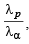to the nearest integer, is                   (2010)

Ans. 3

Solution.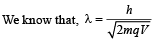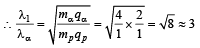Q.2. To determine the half life of a radioactive element, a student plots a graph of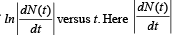is the rate of radioactive decay at time t. If the number of radioactive nuclei of this element decreases by a factor of p after 4.16 years, the value of p is                   (2010)

Ans. 8

Solution. We know that N = N0e-λt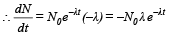Taking log on both sides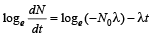Comparing it with the graph line,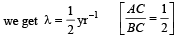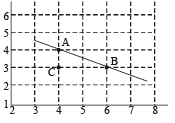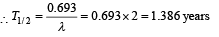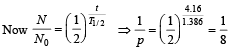Q.3. The activity of a freshly prepared radioactive sample is 1010 disintegrations per second, whose mean life is 109 s. The mass of an atom of this radioisotope is 10–25 kg. The mass (in mg) of the radioactive sample is                        (2011)

Ans. 1

Solution.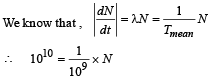∴ N = 1019

i.e. 1019 radioactive atoms are present in the freshly prepared sample.

The mass of the sample = 1019 × 10–25 kg = 10–6kg = 1 mg

Q.4. A silver sphere of radius 1 cm and work function 4.7 eV is suspended from an insulating thread in freespace. It is under continuous illumination of 200 nm wavelength light. As photoelectrons are emitted, the sphere gets charged and acquires a potential. The maximum number of photoelectrons emitted from the sphere is A × 10z (where 1 < A < 10). The value of  ‘z’ is       (2011)

Ans. 7

Solution. Stopping potential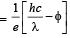where hc = 1240eV –nm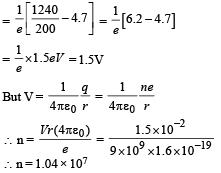Comparing it with A × 10z we get,   z = 7

Q.5. A proton is fired from very far away towards a nucleus with charge Q = 120 e, where e is the electronic charge.

It makes a closest approach of 10 fm to the nucleus. The de Broglie wavelength (in units of fm) of the proton at its start is: (take the proton mass, m= (5/3) × 10 – 27 kg; h/e = 4.2 × 10–15 J.s / C;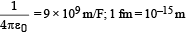(2012- I)

Ans. 7

Solution. Loss in K.E. of proton = Gain in potential energy of the proton – nucleus system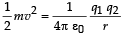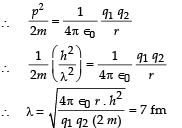Q.6. The work functions of Silver and Sodium are 4.6 and 2.3 eV, respectively. The ratio of the slope of the stopping potential versus frequency plot for Silver to that of Sodium is         (JEE Adv. 2013-I)

Ans. 1

Solution. For photoelectric effect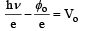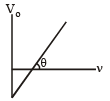The slope is

tan θ = h/e = constant

∴ The ratio will be 1.

Q.7. A freshly prepared sample of a radioisotope of half-life 1386 s has activity 103 disintegrations per second. Given that ln2 = 0.693, the fraction of the initial number of nuclei (expressed in nearest integer percentage) that will decay in the first 80 s after preparation of the sample is                (JEE Adv. 2013-I)

Ans. 4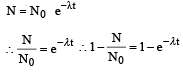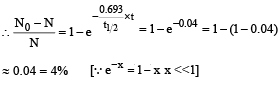Q.8. A nuclear power plant supplying electrical power to a village uses a radioactive material of half life T years as the fuel. The amount of fuel at the beginning is such that the total power requirement of the village is 12.5% of the electrical power available from the plant at that time. If the plant is able to meet the total power needs of the village for a maximum period of nT years, then the value of n is                   (JEE Adv. 2015)

Ans. 3

Solution.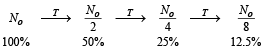Three half life are required. Therefore n = 3

Q.9. Consider a hydrogen atom with its electron in the nth orbital.

An electromagnetic radiation of wavelength 90 nm is used to ionize the atom. If the kinetic energy of the ejected electron is 10.4 eV, then the value of n is (hc = 1242 eV nm)             (JEE Adv. 2015)

Ans. 2

Solution.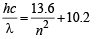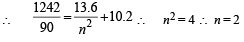Q.10. For a radioactive material, its activity A and rate of change of its activity R are defined as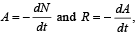where N(t) is the number of nuclei at time t. Two radioactive sources P (mean life τ) and Q (mean life 2τ) have the same activity at t = 0. Their rates of change of activities at t = 2τ are RP and RQ, respectively.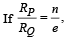then the value of n is                    (JEE Adv. 2015)

Ans. 2

Solution.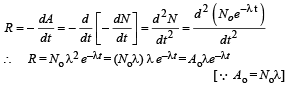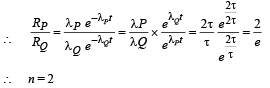Q.11. An electron is an excited state of Li2+ ion has angular momentum 3h/2π. The de Broglie wavelength of the electron in this state is pπ a0 (where a0 is the Bohr radius). The value of p is                   (JEE Adv. 2015)

Ans. 2

Solution. Given mvr = 3h/2π ⇒ n = 3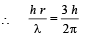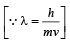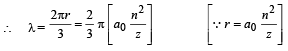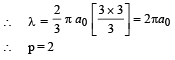Q.12. The isotope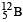having a mass 12.014 u undergoes β–decay to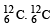has an excited state of the nucleus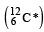at 4.041 MeV above its ground state.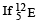decays to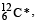the maximum kinetic energy of the β–particle in units of MeV is (1 u = 931.5 MeV/c2, where c is the speed of light in vacuum).           (JEE Adv. 2016)

Ans. 9

Solution. Maximum kinetic energy of β-particle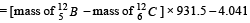= [12.014 – 12] × 931.5 – 4.041] = 9MeV

Q.13. A hydrogen atom in its ground state is irradiated by light of wavelength 970 Å. Taking hc/e = 1.237 × 10–6 eV m and the ground state energy of hydrogen atom as –13.6 eV, the number of lines present in the emission spectrum is        (JEE Adv. 2016)

Ans. 6

Solution.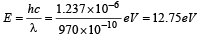∴ The energy of  electron after absorbing this photon

= –13.6 + 12.75 = – 0.85eV

This corresponds to n = 4

Number of spectral line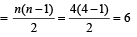Offer running on EduRev: Apply code STAYHOME200 to get INR 200 off on our premium plan EduRev Infinity!

42 docs|19 tests

,

,

,

,

,

,

,

,

,

,

,

,

,

,

,

,

,

,

,

,

,

,

,

,

,

,

,

,

,

,

;